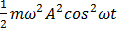# A particle undergoing simple harmonic motion has time dependent displacement given by x(t)=Asin. The ratio of kinetic to potential energy of this particle at t=210 s will be:

## Question ID - 50307 :- A particle undergoing simple harmonic motion has time dependent displacement given by x(t)=Asin. The ratio of kinetic to potential energy of this particle at t=210 s will be:

3537

k=U =cot2cot2=Hence ratio is 3 (most appropriate)

Next Question :
 Ice at −20°c is added to 50 g of water at 40° c. when the temperature of the mixture reaches 0°c, it is found that 20 g of ice is still un melted. The amount of ice added to the water was close to (specific heat of water =4.2 J/g/°c) Specific heat of Ice=2.1 J/g/°C Heat of fusion of water at 0°C = 334 J/g) (a) 50g (b) 40g (c) 60g (d) 100g